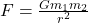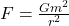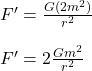## Two asteroids are located a certain distance away from each other. They have the same mass . The gravitational force of attraction is f . If

Question

Two asteroids are located a certain distance away from each other. They have the same mass . The gravitational force of attraction is f . If the mass of one of the asteroids is doubled, how will the gravitational force be affected

in progress 0
2 months 2021-07-29T22:35:59+00:00 1 Answers 1 views 0

F’ = 2F

Explanation:

The gravitational force of attraction between asteroids is given by Newton’s Law of Gravitation:where,

F = Force

G = Universal Gravitational Constant

m₁ = m₂ = m = mass of each asteroid

r = distance between asteroids

Therefore,——————— eq(1)

Now, if we double the mass of one asteroid:using equation (1):

F’ = 2F